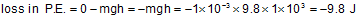Request a call back

sir i hve a question. a raindrop of mass 1gm falling from a height of 1km hits the ground with a speed of 50m/s.calculate- 1. loss of potential energy of drop 2.gain in K.E of drop 3.is gain in kinetic energy equal to loss of potential energy?if not why??? plz reply as soon as possible sir!!!
Asked by shravan s | 07 Oct, 2013, 05:55: PMExpert Answer
Mass of raindrop is = m = 1 gm = 1 × 10-3 kg.
Height from where the raindrop falls is h = 1 km = 1 × 103 m.

1) Now, loss in potential energy is2) Now, gain in kinetic energy is3) The loss in potential energy is not equal to the gain in kinetic energy. In ideal case the raindrop should have hit with a greater speed.
This is because, part of the energy is lost in overcoming air resistance and part goes as sound after hitting the ground.
Answered by Romal Bhansali | 08 Oct, 2013, 12:19: PM